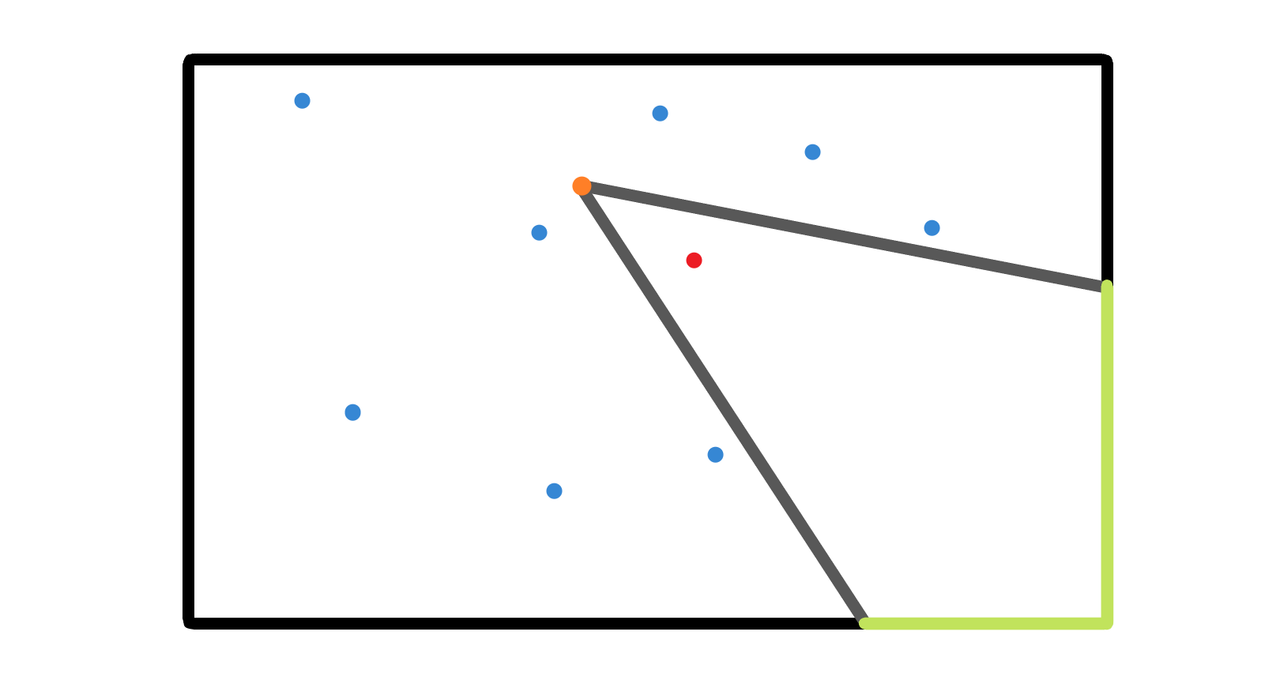## C Cameraman## K Kingdom’s Power

int dfs(int pos = 1, int dep = 0) {
if (ch[pos].empty()) {
ans += dep;
return 1;
}
int height = 0;
bool flag = 1;
for (auto p : ch[pos]) {
auto tmp = dfs(p, dep + 1);
height = max(height, tmp);
if (tmp < dep)
ans -= dep - tmp;
else
flag = 0;
}
if (flag) ans += dep - height;
return height + 1;
}